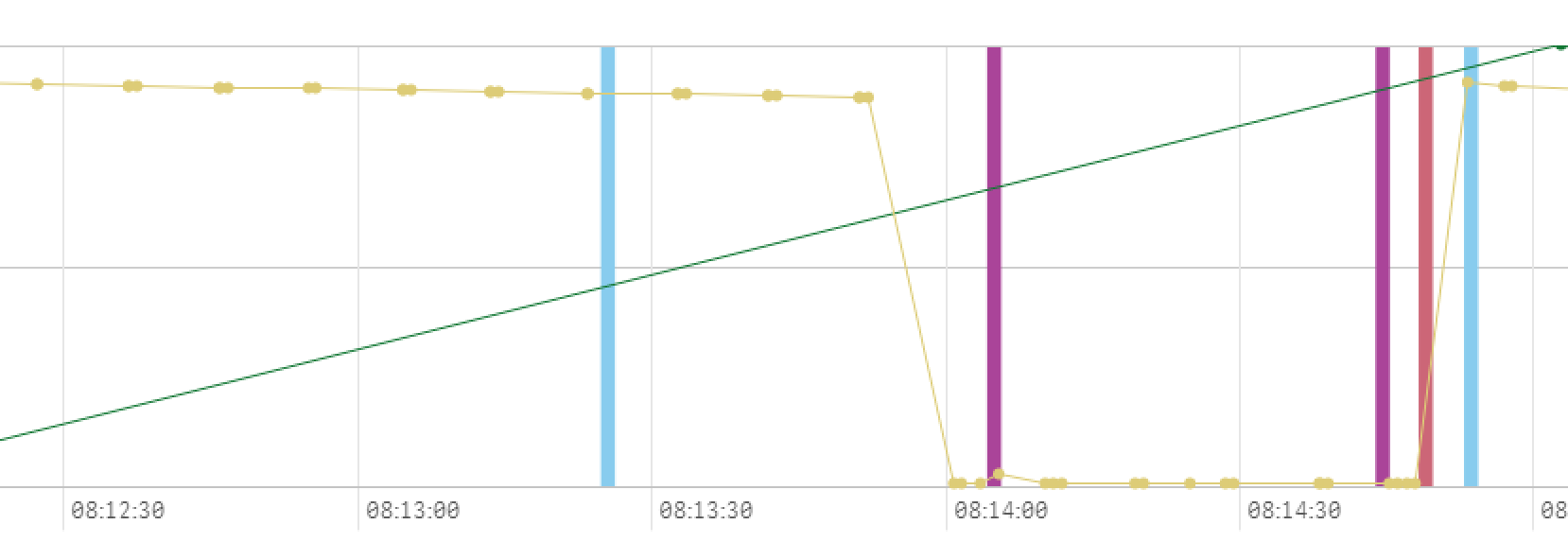# QlikView Scripting

Discussion Board for collaboration on QlikView Scripting.

Announcements

#### Breathe easy -- you now have more time to plan your next steps with Qlik! QlikView 11.2 Extended Support is now valid through December 31, 2020. Click here for more information.

New Contributor III

## Sum element from one table divided by time interval from another.

Hi,

I have two tables in my qlik sense applications.

[table_one]:

[index],

[Value],

[CicleId],

[CicleEnd],

[CicleStart],

[time_stamp];

[table_two]:

[id],

[diIndex],

[diStatus],

[time_stamp];

I try to create table where I would have dimension: CicleId, and measurement sum of diStatus for this cicle period.

What I did is

Sum({<table_two.time_stamp={">=table_one.CicleStart"},table_two.time_stamp={"<=table_one.CicleEnd"},table_two.diIndex={15}>}diStatus)

But What I get is for all rows 0 but in total I get some value (145)

How to fix it

Tags (2)
9 RepliesMVP

## Re: Sum element from one table divided by time interval from another.

Perhaps this? I am assuming, script involves Qualify statement.

Sum({<[table_two.time_stamp]={"=[table_two.time_stamp] >= [table_one.CicleStart]"},[table_two.time_stamp]={"=[table_two.time_stamp] <= [table_one.CicleEnd]"},table_two.diIndex={15}>}diStatus)

Life is so rich, and we need to respect to the life !!!
New Contributor III

## Re: Sum element from one table divided by time interval from another.

No, this is still not correct answer. I think you assume, that timestamp in one table and timestamp in second are the same, but they are notMVP

## Re: Sum element from one table divided by time interval from another.

FYI, This should work. But if it won't work that means your data model may effect to load the data points. Will you able to provide sample

Life is so rich, and we need to respect to the life !!!
New Contributor III

## Re: Sum element from one table divided by time interval from another.

I am not sure what you mean by provide sample. I already provide, how tables look like.

I can provide screenshots of my application.I am sum-ing the purple lines.As you can see, in range from 8.09 to 8.14.50 I have two lines, so the sum should be 2.

Also for next period from 8.14.50 to 8.20.12  I have two lines as well.MVP

## Re: Sum element from one table divided by time interval from another.

Means, You are just sharing layout of schema not the input values. Some where i would think this has format issue

I apologies to review is not clear from my end, It should be like below

Sum({<[table_two.time_stamp]={"=[table_two.time_stamp] >= [table_one.CicleStart] and [table_two.time_stamp] <= [table_one.CicleEnd]"},table_two.diIndex={15}>}diStatus)

Life is so rich, and we need to respect to the life !!!
New Contributor III

## Re: Sum element from one table divided by time interval from another.

You are right, there could be a format issue.

I convert it to

Sum({<[table_two.time_stamp]={"=[table_two.time_stamp] >= [Date(table_one.CicleStart, 'YYYY-DD-MM HH:mm:ss')] and [table_two.time_stamp] <= [Date(table_one.CicleEnd, 'YYYY-DD-MM HH:mm:ss')]"},table_two.diIndex={15}>}diStatus)

but it is still not working.MVP

## Re: Sum element from one table divided by time interval from another.

What is the format for

1) [table_two.time_stamp]

2) [table_one.CicleStart]

3) [table_one.CicleEnd]

Life is so rich, and we need to respect to the life !!!MVP

## Re: Sum element from one table divided by time interval from another.

I have a feeling that you might need to use if statement rather than using set analysis here.... but I am not 100% sure... can you share some sample data in an Excel file and explain the output you are trying to get?

New Contributor III

## Re: Sum element from one table divided by time interval from another.

hmmm,... I don't know exactly how to look for that.

The time_stamp is a field from postgres database field TimeStamp.

In Table it is formatted as : 2017-11-06 10:28:40.380000

The CicleStart and End are derivates from time_stamp, like: